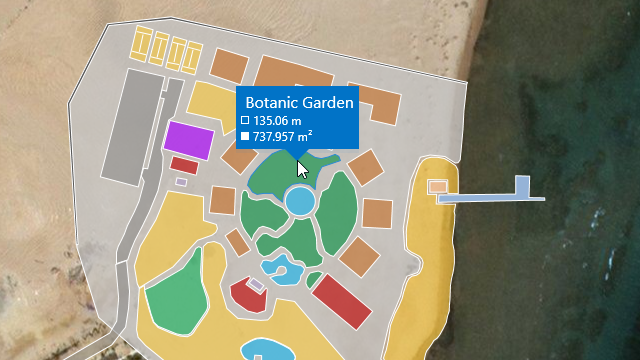# GeoUtils Class

Cartography measurement API for maps with a geographical coordinate system.

Namespace: DevExpress.Xpf.Map

Assembly: DevExpress.Xpf.Map.v22.1.dll

## Declaration

``public static class GeoUtils``

## Remarks

The `GeoUtils` class contains methods that allow you to calculate geometric values (for example, a shape perimeter or area) based on geographical coordinates.The following list contains the main `GeoUtils` methods:

• CalculateArea(MapShape shape) computes a map shape’s total area, in square meters.

``````private void MapControl_SelectionChanged(object sender, MapItemSelectionChangedEventArgs e) {
MapShape mapShape = vectorLayer.SelectedItems as MapShape;
double areaByShape = GeoUtils.CalculateArea(mapShape) / 1000000;
label.Content = \$"Shape Area: {areaByShape:F2} km²";
}
``````
• CalculateArea(IList<GeoPoint> points) calculates an area of the region given by geographical points, in square meters.

``````double areaByPoints = GeoUtils.CalculateArea(new List<GeoPoint> {
new GeoPoint(41, -109),
new GeoPoint(41, -102),
new GeoPoint(37, -102),
new GeoPoint(37, -109)
});
``````
• CalculateBearing(GeoPoint p1, GeoPoint p2) computes an angle between the Geodetic North direction and a line given by two points, clockwise in degrees.

``````double bearing = GeoUtils.CalculateBearing(new GeoPoint(36.1, -115.1), new GeoPoint(35.9, -115.9));
``````
• CalculateCenter(GeoPoint p1, GeoPoint p2) finds a center point between two geographical points.

``````GeoPoint centerPoint = GeoUtils.CalculateCenter(
new GeoPoint(35.286, -111.1724),
new GeoPoint(38.3802, -110.9307));
``````
• CalculateDistance(GeoPoint p1, GeoPoint p2) determines the distance between two geographical points in meters. The ellipsoidal model of the Earth is used in the calculations of the distance.

``````double distance = GeoUtils.CalculateDistance(new GeoPoint(36.1, -115.1), new GeoPoint(34, -118.2));
``````
• CalculateDistance(GeoPoint p1, GeoPoint p2, Boolean ignoreEllipsoidalEffects) determines the distance between two geographical points in meters. This `CalculateDistance` method overload allows you to specify whether to use the ellipsoidal-surface formula or spherical-surface formula to calculate the distance.

``````using DevExpress.Xpf.Map;

private void OnButtonClick(object sender, RoutedEventArgs e) {
double distance = GeoUtils.CalculateDistance(new GeoPoint(-5.93107, -35.112723),
new GeoPoint(4.253438, 5.47072), true);
}
``````
• CalculateDestinationPoint(GeoPoint startPoint, Double distance, Double bearing) calculates a geographical point based on the specified start point, distance, and bearing. The distance is measured along a geodesic line.

``````GeoPoint startPoint = new GeoPoint(53.1914, 001.4347);
GeoPoint destinationPoint = GeoUtils.CalculateDestinationPoint(startPoint, 17910000, 276.0117);
``````
• CalculateLength(IList<GeoPoint> points) calculates the polyline length, in meters.

``````double lineLength = GeoUtils.CalculateLength(new List<GeoPoint> {
new GeoPoint(35.286, -111.1724),
new GeoPoint(38.3802, -110.9307),
new GeoPoint(36.9365, -102.493)
});
``````
• CalculateStrokeLength(MapShape shape) returns the map shape’s stroke length, in meters.

``````private void MapControl_SelectionChanged(object sender, MapItemSelectionChangedEventArgs e) {
MapShape mapShape = vectorLayer.SelectedItems as MapShape;
double strokeLength = GeoUtils.CalculateStrokeLength(mapShape);
label.Content = \$"StokeLength: {strokeLength / 1000:F2} km";
}
``````

Object
GeoUtils